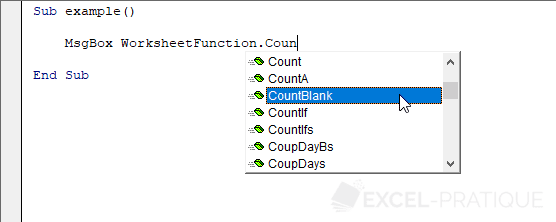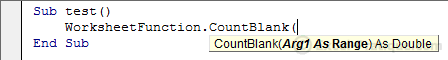# VBA Course: Using Excel functions

It is also possible to use Excel functions in VBA code, as we will see on this page.

After we have entered WorksheetFunction, the list of functions will appear:Choose the function that you are interested in using, for example, COUNTBLANK:In the following macro, the number of empty cells in the "A1:D8" range is stored in a variable and then displayed in a dialog box:

``````Sub test()
var_test = WorksheetFunction.CountBlank(Range("A1:D8"))
MsgBox var_test
End Sub``````